Study Guide

So, you already know about functions. They sit there, looking all y = f(x), showing a relationship between the variables x and y. We also have this quadratic equation just sitting here, not doing anything. How about we mash these two concepts together, all Frankenstein-style?With a dramatic crash of thunder, we have:

f(x) = ax2 + bx + c

A quadratic function. Dun dun dunnnn.

What do we do with functions? We graph them, of course.

### Sample Problem

Graph the quadratic function f(x) = x2.

We start off by finding a few ordered pairs and putting them in a table.

 x f(x) 0 0 1 1 -1 1 2 4 -2 4 3 9 -3 9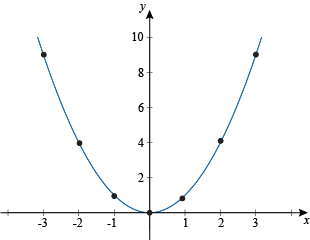By finding even more points, we could show that the graph will continue to curve upwards, reaching larger and larger values of y for the next value of x. In fact, we'll show that with our next…

### Sample Problem

Graph the quadratic function f(x) = -2x2 + x + 10.

Again we start off by finding a few points to get an idea about the general shape of the graph.

 x f(x) 0 10 1 9 -1 7 2 4 -2 0 3 -5 -3 -11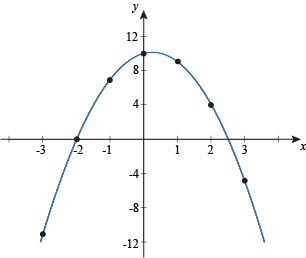Now this graph looks similar to the previous one, but it's curved down. Both of these graphs, and all others made by quadratic functions, are called parabolas. In addition to their good looks, all parabolas share several other common features.

• They have a single maximum or minimum point, called the vertex. The first graph above has a minimum and always increases in both directions away from the vertex, while the second graph has a maximum and is always decreasing.

• Parabolas also have good posture, so they're always symmetric across some vertical line x = h, with the axis of symmetry passing through the vertex (h, k).

• All parabolas have one y-intercept, but parabolas can have 0, 1, or 2 x-intercepts.

### Sample Problem

Graph the quadratic function f(x) = -x2 + 8x – 10.

Another day, another table.

 x f(x) 0 -10 1 -3 -1 -19 2 2 -2 -30 3 5 -3 -43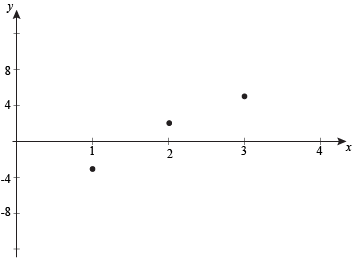That…doesn't look too good. This is a quadratic function, so we know that the graph should look like a parabola. We must be missing some crucial points. As x becomes more negative, the values of y become lower and lower, so the vertex must be to the right of what we have already. Some experimentation finds:

 x f(x) 4 6 5 5 6 2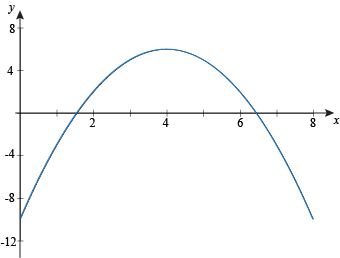That looks better. In the next few sections, we'll learn some handy tricks for graphing parabolas that'll make things loads easier.

• ### Vertex Form Parabolas

The difficulty of graphing a quadratic function varies depending on the form you find it in. We'll start things off relatively easily.

f(x) = a(xh)2 + k

No, we're not lying to you; that is a quadratic function. Go ahead, multiply it out.

f(x) = ax2 + (-2ah)x + (ah2 + k

The h and k are constants, so (-2ah) and (ah2 + k) are also constants, which we could call, say, b and c. See, you can trust us, it's totally quadratic.

When you have a parabola written out like f(x) = a(xh)2 + k, it's in vertex form. With vertex form, you have several pieces of important information thrown at you right away. When you first meet someone, your first impression tends to stick with you. It's the same with this equation.

The sign of a tells you if the parabola opens up or down. If a is positive, it opens up. If a is being a Negative Nancy, the parabola opens down. You also know that the vertex of the parabola is at the point (h, k). Be careful with the sign of h, though.

### Sample Problem

Graph the function f(x) = (x – 2)2 – 1.

The vertex of the parabola is at (h, k) = (2, –1). We can also see that the parabola opens upwards. We need a few more points, however. We could make a table and start plugging in values of x, but there's usually an easier way: find the intercepts of y and x (if they exist). Starting with the y-intercept, which occurs at x = 0.

f(0) = (0 – 2)2 – 1 = 4 – 1 = 3

Right on: (0, 3) is a point on our parabola. Now go for the x-intercepts, which occur when y = 0, if there are any.

0 = (x – 2)2 – 1

0 = x2 – 4x + 4 – 1

0 = x2 – 4x + 3

This quadratic equation can be factored.

0 = (x – 3)(x – 1)

So, (1, 0) and (3, 0) are also points on the parabola. Putting it all together gets us: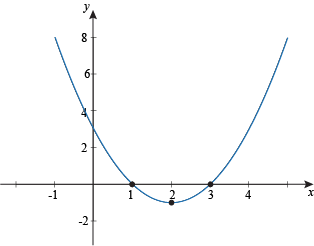See? Math smarter, not harder.

### Sample Problem

Graph the function f(x) = -2(x + 1)2 – 2.

We immediately see that the vertex is at (-1, -2), and the parabola opens down. Time to track down our y-intercept.

f(0) = -2(0 + 1)2 – 2 = -2(1) – 2 = -4

Sweet. The y-intercept is (0, -4). Now we hunt for the x-intercepts. Some say the use of dynamite while hunting is unsportsmanlike. They're probably right.

0 = -2(x + 1)2 – 2

0 = -2(x2 + 2x + 1) – 2

0 = -2x2 – 4x – 2 – 2

0 = -2x2 – 4x – 4

0 = -x2 – 2x – 2

At this point, we hit a wall. The discriminant of this equation is:

b2 – 4ac =(-2)2 – 4(-1)(-2) = 4 – 8 = -4

That's negative, so there are no real roots for this equation. This means that the function will never cross the x-axis, and so there are no x-intercepts. This makes sense given that the vertex is at (-1, -2) and the parabola points down, so the function won't go up towards the x-axis. Guess we won't need that dynamite after all.

Instead of using the x-intercepts, we'll plug in a few extra values of x and plot those.

 x f(x) -3 -10 -4 -20

We're almost ready to finish off this graph. This will be easier to do with a few more points, though. Remember that the axis of symmetry passes through the vertex; we can use this to find several more points now, since we have points on both sides of the vertex.

Compare (0, -4) to the vertex at (-1, -2), for instance. It's 1 to the right on the x-axis and 2 lower on the y-axis. Because the function is symmetric, 1 space to the left of the vertex will also be 2 lower on the y-axis, at (-2, -4). Similarly, (-3, -10) is 2 spaces to the left of the vertex and 8 down, and (-4, -20) is 3 spaces left and 18 spaces down. Reflect those onto the right side of the vertex and we'll see (1, -10) and (2, -20) are also points on the parabola. Now we can confidently graph this bad boy.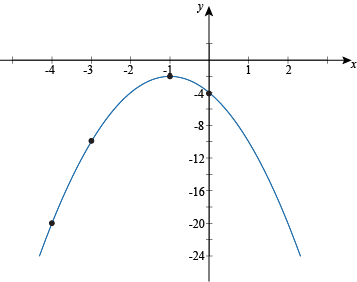### Vertex Parabola Strategery

When you find yourself graphing a parabola in vertex form, here's what you do.

• Check the sign of a to see if it opens up or down.

• Find the vertex and the y-intercept.

• Determine if there are any x-intercepts, either by comparing the shape of the parabola and the vertex, or by checking the discriminant of the expanded function.

• Find the x-intercepts, if they exist.

• Check if you have enough points to finish the graph. If so, hurray.

• If not, boo. Plot a few more points, and use the function's symmetry to find more points.

• Finish up and call it a day.

The vertex form for parabolas is convenient for graphing, but oftentimes you'll be given the equation in the usual quadratic form instead.

f(x) = ax2 + bx + c

Can we deduce a way to find the vertex of a parabola from its quadratic form equation? Would we even ask the question if the answer was "No?"

f(x) = a(xh)2 + k
f(x) = ax2 + (-2ah)x + (ah2 + k

Remember? We did this in the last section. It wasn't that long ago. Anyway, if you compare the second function above to the standard quadratic function, you'll notice that -2ah = b. And hey—if we're given a quadratic function, we know what a and b are just from lookin' at the equation. That means we can solve for h without batting an eyelash.

-2ah = b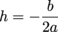While we could go ahead and solve c = ah2 + k for k, there's a much, much better way. Since h is a value of x just like any other, we can just plug h into the function and solve. That means that the vertex equals (h, f(h)), or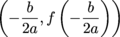. Yep, that's way easier. Smarter, not harder, remember?

### Sample Problem

Graph the function f(x) = 4x2 + 6x – 2.

Let's start off by looking for the vertex. Using our handy-dandy new formula, we find: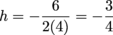Next we plug h in for x to find y. Golly, that's a lot of variables.So the vertex is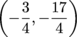. By the way, the rule about the a-value telling us which direction our parabola opens? Yeah, that still applies. Since a is positive 4 in this case, our parabola opens upward.

The y-intercept is easy to find; just track down f(0):

f(0) =4(0)2 + 6(0) – 2 = -2

That puts our y-intercept at (0, -2).

Next we'll check to see if any x-intercepts exist. The discriminant is b2 – 4ac = 62 – 4(4)(-2) = 36 + 32 = 68. That's positive, so we have two real number roots, a.k.a. two x-intercepts. Quadratically formulating a solution, we get: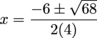Notice that we already know what goes under the square root, because we calculated the discriminant.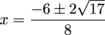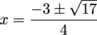Bam: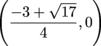and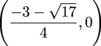are our x-intercepts, which are equal to about (0.281, 0) and (-1.781, 0).

These are enough points for us to graph the function, so let's do that.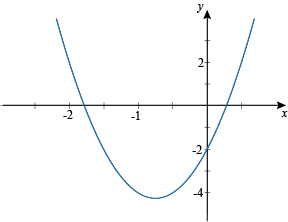When our function is in quadratic form, we pretty much follow the same steps we did in vertex form: find the vertex, find the intercepts, and hunt down any extra points we need to round things out.